Home > A2C > Chapter 7 > Lesson 7.3.3 > Problem7-187

7-187.
1. Perform each of the following matrix operations without using a calculator. If the operation isimpossible, explain why. Homework Help ✎

1.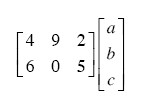2.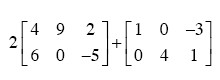3.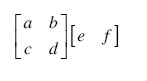4.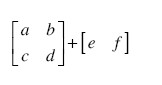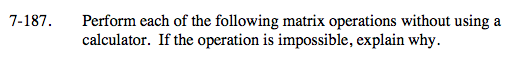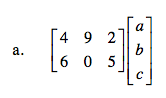What have you learned about matrix operations so far?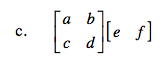What must be true about the dimensions of two matrices in order to multiply them?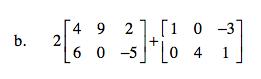Multiply through by the 2 before you add.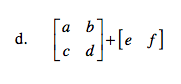What must be true about the dimensions of two matrices in order to add them?